Question

the ratio of the length to the width of a rectangle is 10:6. if the perimeter of the rectangle is 160 meters, find the measure of the width.

1.bonexptip

Given:

The ratio of the length to the width of a rectangle is 10:6.

Perimeter = 160 meters

To find:

The measure of the width.

Solution:

Let the length and width of the rectangle be 10x and 6x respectively.

Perimeter of a rectangle is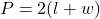where, l is length and w is width.

Putting P=160, l=10x and w=6x, we get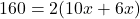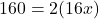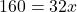Divide both sides by 32.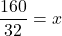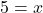Now,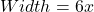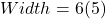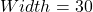Therefore, the width of the rectangle is 30 meters.